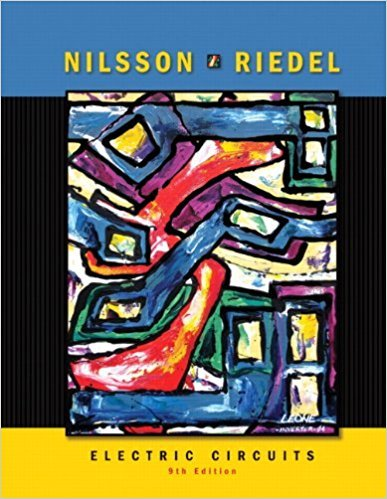×
×

# For the circuit shown, a) If vg = 1 kV and ig = 5 mA, find the value of R and the powerISBN: 9780136114994 391

## Solution for problem 2.3 Chapter 2

Electric Circuits | 9th Edition

• Textbook Solutions
• 2901 Step-by-step solutions solved by professors and subject experts
• Get 24/7 help from StudySoup virtual teaching assistantsElectric Circuits | 9th Edition

4 5 1 430 Reviews
18
2
Problem 2.3

For the circuit shown, a) If vg = 1 kV and ig = 5 mA, find the value of R and the power absorbed by the resistor. b) If ig - 75 mA and the power delivered by the voltage source is 3 W, find vg, R, and the power absorbed by the resistor. c) K JR. 300 ft and the power absorbed by R is 480 mW, find L and vg.

Step-by-Step Solution:
Step 1 of 3

ENGR 103 Lecture 19 Resistance- the ability of an object to restrict or resist the flow of electrons in an electrical circuit - measured in ohms (Ω) Resistor- the circuit element responsible for restricting the flow of electrons - absorb energy from the electrons and dissipate it as heat - since there is a transfer of energy through a resistor,...

Step 2 of 3

Step 3 of 3

##### ISBN: 9780136114994

Electric Circuits was written by and is associated to the ISBN: 9780136114994. Since the solution to 2.3 from 2 chapter was answered, more than 347 students have viewed the full step-by-step answer. This textbook survival guide was created for the textbook: Electric Circuits, edition: 9. The answer to “For the circuit shown, a) If vg = 1 kV and ig = 5 mA, find the value of R and the power absorbed by the resistor. b) If ig - 75 mA and the power delivered by the voltage source is 3 W, find vg, R, and the power absorbed by the resistor. c) K JR. 300 ft and the power absorbed by R is 480 mW, find L and vg.” is broken down into a number of easy to follow steps, and 72 words. The full step-by-step solution to problem: 2.3 from chapter: 2 was answered by , our top Engineering and Tech solution expert on 03/13/18, 07:50PM. This full solution covers the following key subjects: . This expansive textbook survival guide covers 18 chapters, and 1109 solutions.

Unlock Textbook Solution